## Brief introduction to SimPy¶

SimPy is a process-based discrete-event simulation framework based on Python.

The behavior of active components (like vehicles, customers or messages) is modeled with processes. All processes live in an environment. They interact with the environment and with each other via events.

First, check if simpy is installed, otherwise use the following line for a quick install:

In :
!pip install simpy

Requirement already satisfied: simpy in /opt/anaconda3/lib/python3.8/site-packages (4.0.1)

In :
import simpy
import matplotlib.pyplot as plt


### Car parking example¶

We will create a car process that has two possible actions: drive and park.

Processes are described by Python generators. During their lifetime, they create events and yield them in order to wait for them to be triggered.

An important event type is the timeout. Events of this type are triggered after a certain amount of (simulated) time has passed. They allow a process to sleep (or hold its state) for the given time.

In our car parking example, the two actions (drive and park), are defined as events with different timeout durations.

In :
 def car(env):
while True:
print('Start parking at %d' % env.now)
parking_duration = 5
yield env.timeout(parking_duration)

print('Start driving at %d' % env.now)
trip_duration = 2
yield env.timeout(trip_duration)


Once we have created the car process, we must define a simuation environment and run the process for a certain amount of time.

Events within the process will be yielded consecutively until time runs out.

In :
env = simpy.Environment()
env.process(car(env))
env.run(until=30)

Start parking at 0
Start driving at 5
Start parking at 7
Start driving at 12
Start parking at 14
Start driving at 19
Start parking at 21
Start driving at 26
Start parking at 28


Different processes can interact within the same environment, giving rise to complex simulation models.

We encourage you to look for additional information regarding SimPy and it's different uses in the official documentation:

Now, we will use a model based on SimPy to approach an Inventory Management problem.

## Problem Statement¶

A company that sells a single product would like to decide how many items it should have in inventory for each of the next n months. The times between demands are IID exponential random variables with a mean of 0.1 month. The sizes of the demands, D, are IID random variables (independent of when the demands occur), with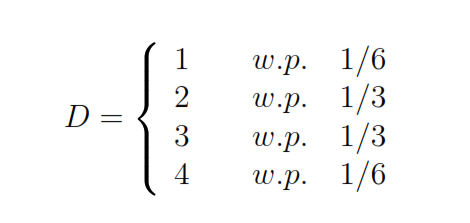At the beginning of each month, the company reviews the inventory level and decides how many items to order from its supplier. If the company orders $Q$ items, it incurs a cost of $K + iZ$, where $K= \unicode{163} 32$ is the setup cost and $i=\unicode{163}3$ is the incremental cost per item ordered (if $Q=0$, no cost is incurred). When an order is placed, the time required for ir to arrive (called the lag or lead time) is a random variable that is distributed uniformly between 0.5 and 1 month.

The company uses a stationary (s, S) policy to decide how much to order i.e.,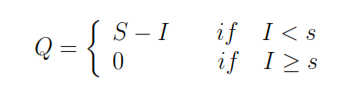where I is the inventory level at the beginning of the month. When a demand occurs, it is satisfied immediately if the inventory level is ar least as large. If the demand exceeds the inventory, the difference is backlogged and satisfied in future deliveries.

In addition to the ordering costs, we will consider two other costs:

• Holding cost $h$: this includes such costs as warehouse rental, insurance, taxes and maintenance. We will assume $\unicode{163} 1$ per item held in inventory per month.
• Shortage cost $\pi$: this accounts for the cost of extra record keeping when a backlog exists as well as loss of customers' goodwill. We will assume $\unicode{163} 5$ per item in backlog per month.

We want to compare different values for the policy (s,S) in a simulated environment, for a period of 120 months. The values for (s,S) can be found below. Assume the initial inventory is set to 60.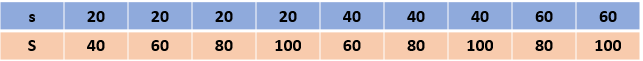### SimPy Model¶

The complete model for this problem is in the file model.py. As you can see, it is composed of three SimPy processes that interact with each other within the same environment:

• check_inventory: Checks inventory at regular interval and places order if needed
• place_order: Places order and updates inventory level when order has been received
• demand: Generates demand at random intervals and updates inventory level

In addition, the function update_costs calculates shortage and holding costs before each change in inventory.

In :
import numpy as np
import model
import matplotlib.pyplot as plt


To run a single iteration of the inventory simulation model, use the 'run' function of the model.py file. Users need to enter:

• a simulation length (expressed in days) after which the simulation stops
• a reorder point specifying the inventory level (in units) that triggers an order to replenish the stocks
• an order size specifying the number of units to order

The function will return a dictionary containing user input parameters (reorder point, order size) and the model output (average monthly total inventory cost, ordering cost, shortage cost and holding cost).

Let's run a few iterations and have a look at their output. Note that the model contains random variables (delivery delay and demand size and intervals), therefore different results will be obtained by independent runs.

s = reorder_point

S = reorder_point + order_size

In :
# perform a single run of the inventory simulation model
results, history = model.run(length = 120., reorder_point = 20, order_size = 20)

In :
results

Out:
{'reorder_point': 20,
'order_size': 20,
'total_cost': 124.3,
'ordering_cost': 98.9,
'holding_cost': 9.5,
'shortage_cost': 15.8}

we can look at the inventory history in a bar graph. The negative values correspond to the demand backlog.

In :
fig, ax = plt.subplots(dpi=100)
plt.bar(range(120),history)
plt.xlabel('Month')
plt.ylabel('Inventory level')

Out:
Text(0, 0.5, 'Inventory level')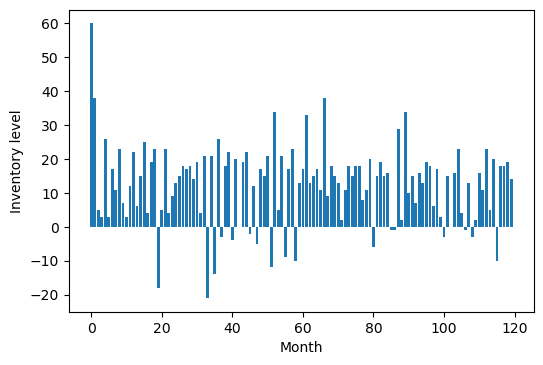### Building a confidence interval for total inventory cost¶

Unless the simulation model is purely deterministic (i.e. there is no randomness in the model), it will be required to conduct a statistical analysis of the simulation output.

Running a single iteration of the simulation and reporting the performance measures is not a recommended approach. As we saw above, independent replications of a simulation will yield different output, which can result in making poor decisions.

By doing several independent replications of each configurations allows to measure the spread of results and build a confidence interval for the average performance of the system.

In :
import pandas as pd

length = 120.
num_replications = 15
reorder_point = 30
order_size = 60

df = pd.DataFrame(model.run_experiments(length, [reorder_point], [order_size], num_replications))
df

Out:
reorder_point order_size total_cost ordering_cost holding_cost shortage_cost
0 30 60 123.0 84.0 36.0 3.1
1 30 60 125.7 86.6 35.1 4.0
2 30 60 120.7 81.2 37.3 2.2
3 30 60 123.1 84.7 34.4 4.0
4 30 60 130.7 90.7 34.4 5.6
5 30 60 125.1 85.0 36.8 3.3
6 30 60 119.3 78.2 36.6 4.5
7 30 60 122.4 83.1 37.6 1.7
8 30 60 122.5 83.7 34.6 4.2
9 30 60 123.5 84.1 36.4 3.0
10 30 60 124.3 84.4 36.0 3.9
11 30 60 123.2 85.0 34.4 3.7
12 30 60 127.6 88.7 35.0 3.8
13 30 60 124.7 86.6 34.5 3.7
14 30 60 126.3 86.4 35.4 4.4

We then need to compute our sample mean and sample standard deviation of the total cost output.

Because our sample size is small (less than 30 observations is often used as a rule of thumb), we'll use a t-statistics (as opposed to a z-statistics) to build our confidence interval.

In :
from scipy import stats

# compute sample mean and standard deviation
mean = df['total_cost'].mean()
std = df['total_cost'].std()

# compute t-statistics for a 90% confidence interval
alpha = 1-.9
tstat = stats.t.ppf(1-alpha/2, num_replications - 1)

# compute confidence interval
error_margin = tstat * std / np.sqrt(num_replications)
lbound = mean - error_margin
ubound = mean + error_margin

print("90 percent confidence interval for monthly inventory cost: [%.1f, %.1f]" % (lbound, ubound))

90 percent confidence interval for monthly inventory cost: [122.9, 125.4]


It is a good practice to build confidence intervals whenever performance indicators need to be assessed.

They add valuable information to the average. If system A yields a performance of (100 +- 1) and B yields (100 +- 15), while on average the performance is the same, B is much riskier than A.

Confidence intervals are also interpretable without deep statistical knowledge, which makes it convenient to communicate with simulation stakeholders.

### Comparing alternative configurations¶

We can now compare the different configurations for the policy (s,S) given in the problem statement.

For each configuration, we will run 30 independent runs and compare the average of the total costs.

In :
s = [20, 20, 20, 20, 40, 40, 40, 60, 60]
S = [40, 60, 80, 100, 60, 80, 100, 80 ,100]

num_rep = 30

total_cost_runs = []
total_cost = []
ordering_cost_runs = []
ordering_cost = []
holding_cost_runs = []
holding_cost = []
shortage_cost_runs = []
shortage_cost = []

for j,k in zip(s,S):
for i in range(num_rep):

res, _ = model.run(length = 120., reorder_point = j, order_size = k-j)
total_cost_runs.append(res['total_cost'])
ordering_cost_runs.append(res['ordering_cost'])
holding_cost_runs.append(res['holding_cost'])
shortage_cost_runs.append(res['shortage_cost'])

# compute the average for each sample
total_cost.append(np.mean(total_cost_runs))
ordering_cost.append(np.mean(ordering_cost_runs))
holding_cost.append(np.mean(holding_cost_runs))
shortage_cost.append(np.mean(shortage_cost_runs))

df = pd.DataFrame([zip(s,S), total_cost, ordering_cost, holding_cost, shortage_cost])
df = df.T
df.columns = ['(s,S)','Total Cost','Ordering Cost','Holding Cost','Shortage Cost']

In :
fig, ax = plt.subplots(dpi=100)
ax.plot(df['Total Cost'], label='Total Cost', marker='.')
plt.xticks(range(len(s)), df['(s,S)'])
plt.xticks(rotation=45,ha='right')
plt.xlabel('Inventory policy')
plt.ylabel('Total Costs')
plt.legend()
plt.show()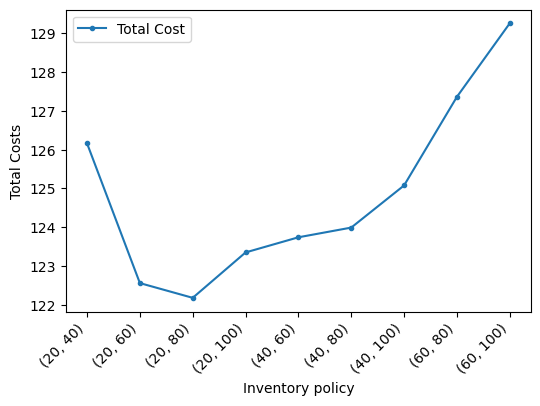We can also look at how the different costs vary for the different configurations.

We notice that increasing S from 40 to 100, increases the holding costs steadily, while reducing the shortage cost.

The effect of the increase in S on the ordering cost is to reduce it, since ordering up to larger values of S implies that these larger orders will be placed less frequently, thereby avoiding the fixed ordering cost more often.

Similarly, fixing S at, say, 100, and increasing s from 20 to 60 leads to a decrease in shortage cost but an increase in holding cost.

In :
df

Out:
(s,S) Total Cost Ordering Cost Holding Cost Shortage Cost
0 (20, 40) 126.16 98.4767 9.02333 18.6633
1 (20, 60) 122.557 93.5667 13.3033 15.695
2 (20, 80) 122.177 90.6856 17.8433 13.6544
3 (20, 100) 123.348 88.76 22.4867 12.1067
4 (40, 60) 123.738 90.5467 23.124 10.074
5 (40, 80) 123.988 90.2789 25.1222 8.59222
6 (40, 100) 125.075 89.6486 27.9152 7.51571
7 (60, 80) 127.36 90.755 30.0242 6.58417
8 (60, 100) 129.256 90.6637 32.7367 5.85741
In :
fig, ax = plt.subplots(dpi=100)
ax.plot(df['Total Cost'], label='Total Cost', marker='.')
ax.plot(df['Ordering Cost'], label='Ordering Cost', marker='.')
ax.plot(df['Holding Cost'], label='Holding Cost', marker='.')
ax.plot(df['Shortage Cost'], label='Shortage Cost', marker='.')
plt.xticks(range(len(s)), df['(s,S)'])
plt.xticks(rotation=45,ha='right')
plt.xlabel('Inventory policy')
plt.ylabel('Costs')
plt.legend()
plt.show()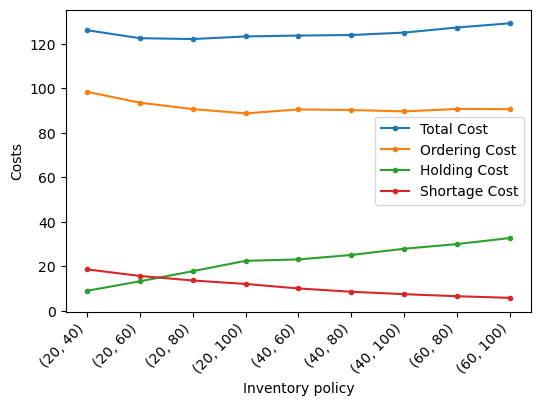In [ ]: